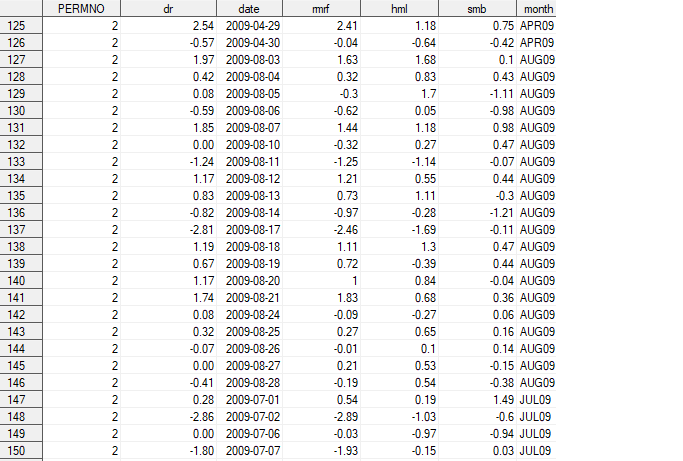## proc optmodel

Hi,

I have the following type of dataset and have as many as 4000 permnos.

I am trying to do the following ,

```min VAR(R.dr - SUM[w_i * R.s_i]) = min VAR(Dr - w*S)
s.t. SUM(w_i) = 1; w_i > 0(R.s_i include rmrf hml smb)```I am using the following code for this. The code works perfectly fine,when I run it for one permno only but I want to run this using a by variable (by permno and month). I am not sure how to incorporate this, I have read this document (http://support.sas.com/kb/42/332.html) but I am not getting how to apply this on my data.

Any help would be much appreciated.

```proc corr data=return1 (drop=date)
out=corrout(where=(_type_ ne 'CORR')) cov noprint;

run;

data stats(drop=_name_);
set corrout;
if _type_ = 'COV' then delete;
run;
proc transpose data=stats out=stats;
id _type_;
run;

proc optmodel;
/* declare parameters and read data */
set <str> ASSETS;
str target = 'f';
set BENCH = ASSETS diff {target};
num cov {ASSETS, ASSETS};
{i in ASSETS} <cov[_name_,i]=col(i)>;
/* let w1, w2, w3, w4 be the amount invested in each asset */
var x{1..4} >= 0;
/* declare optimization model */
var W {BENCH} >= 0 <= 1;
/* Var(X - Y) = Var(X) + Var(Y) - 2 Cov(X,Y) */
min Variance =
sum {i in BENCH, j in BENCH} cov[i,j] * W[i] * W[j]
+ cov[target,target]
- 2 * sum {i in BENCH} cov[i,target] * W[i];
/* subject to the following constraints */
con weights:  sum {i in BENCH} W[i] = 1;

/* call solver and print optimal solution */
solve;
print W;
quit;```